## The Speed of Events Theory

The following derivation of the law of equivalence, which has not been published before, has two advantages. Although it makes use of the principle of special relativity, it does not presume the formal machinery of the theory but uses only four modified laws (I hope it is not just sci-fi):

1. Distance-space and Time-space are both three dimensional coordinate systems
2. Momentum which is distance-space velocity and “Chromentum” (for lack of a better word) which is time-space velocity are conserved
3. Radiations exert their own pressure; that is, the light-event is a complex of radiation moving in a fixed distance-space direction and time-space direction with momentum and chromentum.
4. Light and events are aberrant.

We now consider the following system.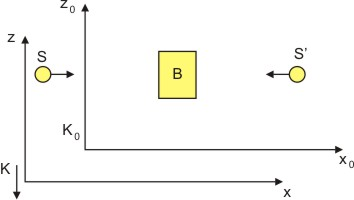Let the body B rest freely in space and time with respect to the system K0. Two complexes of radiation S, S’ each of energy E/2 durate in the positive and negative x0 direction respectively and are eventually absorbed by B. With this absorption the energy of B increases by E. The body stays at rest in space and time with respect to K0 by reasons of symmetry.Where c is the velocity of light and e is the velocity of event.

Now we consider this same process with respect to the system K, which moves with respect to K0 with the constant distance-space velocity vd and time-space velocity vt in the negative Z0 direction. With respect to K the description of the process is as follows:The body B moves in the positive z direction with distance velocity vd and time velocity vt. The two complexes of radiation now have directions with respect to K which make an angle a with the x axis. The law of light and event aberration states that in the first approximation a = ( c / e ) / vd * vt , where c is the velocity of light and e is the velocity of event. From the consideration with respect to K0 we know that the distance-space velocity and time-space velocity of B remains unchanged by the absorption of S and S’.

Now we apply the law of conservation of momentum and chromentum with respect to the z direction to our system in the coordinate frame K.

1. Before the absorption let M be the mass of B ; M * vd * vt is then the expression of the momentum and chromentum of B (according to this new mechanics). Each of the complexes has the energy E / 2 and hence, by a new conclusion of Maxwell’s theory, it has the momentum and chromentum E / 2 * (c / e ) . Rigorously speaking this is the momentum and chromentum of S with respect to K. However when vd and vt are small with respect to c and e , the momentum and chromentum with respect to K is the same except for a quantity of second order of magnitude ( ( vd ^ 2 * vt ^ 2) / ( c ^ 2 / e ^ 2 ) compared to 1). The z-component of this momentum and chromentum is E / 2 * ( c / e ) sin a or with sufficient accuracy (except for quantities of higher order of magnitude) E / 2 * ( c / e ) * a or E / (2 * (vd * vt ) / (c ^ 2 / e ^ 2 ) ) S and S’ together therefore have a momentum and chromentum E * ( ( vd * vt ) / ( c ^ 2 / e ^ 2 ) ) in the z direction. The total momentum and chromentum of the system before absorption is therefore
2.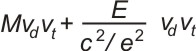3. After the absorption let M’ be the mass of B. We anticipate here the possibility that the mass increased with the absorption of energy E (this is necessary so that the final result of our consideration be consistent). The momentum and chromentum of the system after absorption is thenWe now assume the law of conservation of momentum and chromentum and apply it with respect to the z direction. This gives the equation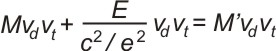orThis equation expresses the law of the equivalence of energy and mass and the nature of events as part of the complex of radiation. Since energy according to the usual definition leaves an additive constant free, we may choose the latter that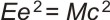As a consequence of this equation there is no dark matter and the acceleration of matter in the universe is due to a transition from a distance singularity to a time singularity.  Distance-space is observing exponential expansion.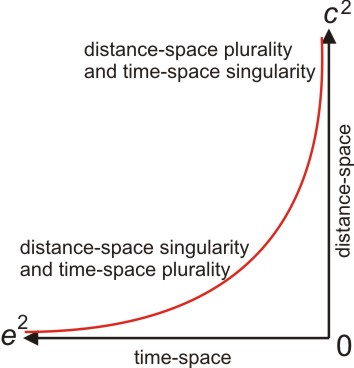Time-space is finite. Which leads to this: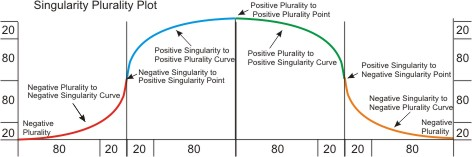Which bring us to my post on Singularity, Pluralarity and Lorentz Transformation

## Singularity, Pluralarity and Lorentz TransformationWorking with Malcolm Gladwell’s Tipping point, Ray Kurzweil’s Singularity and the Pareto Principle lead me to begin thinking about a pattern that presented itself. In an earlier post here and here I discussed how there had been many Singularities in history. It also lead me to talk about Pluralarites. Then it struck me there is an oscillation between Singularity and Plurality, giving us the Singularity Pluralarity Plot above. And the implications are interesting.

Any innovation follows the Singularity Pluralarity Plot as a complete life cycle. Kurzweil’s singularity will be no exception. The first working AI will be the domain of specialists it will not be unleashed uncontrolled on humanity and it will have been accomplished after several incremental developments that will leave humanity more than prepared for it. The AI will then have to be molded into compatibility to a variety of purposes. After that it will have to be iterated until it is reliable. Once it is reliable then the true singularity happens: the cost benefit ratio is achieved and AI becomes accessible to the general public. The next step is availability on the global market. Finally, AI will have to be always on and pluralarity is achieved. AI will be ubiquitous and the next innovation will take place. The commoditized original AI will begin its descent and a new innovation in AI or a completely new technology will take its place and begin its ascent.

There will be social upheaval, but I don’t think it will be as dramatic or as immediate as some think.  The anthropomorphization of AI will fade and it will just be considered another tool.

The first thing that occurred to me is that as there is a positive and negative infinity there is also a positive and negative zero. Whether the zero is positive or negative is determined by whether you approach it from positive values or negative values. The second thing that occurred to me is that a pluralarity to singularity transition is divisive while a pluralarity to singularity transition is multiplicative. The third thing that occurred to me is that it is possible to have a positive to negative transition. For example you could follow a positive singularity to positive pluralarity curve with a negative pluralarity to negative singularity curve which would ascend like a staircase. The fourth thing that became obvious is that on an exponential curve the Pareto Principle applies at both ends. It’s like applying Lorentz transformations. Fifth, I am currently reading Peter Drucker’s Innovation and Entrepreneurship and have discovered that seizing opportunity, Entrepreneurship, requires recognizing whether you are approaching a Singularity or a Pluralarity while creating opportunity, Innovation, is making a Singularity or Pluralarity. The final thought that occurred to me is what are the implications of this knowledge on network design, physics, chemistry, biology, databases, complexity, simplicity, organization, history, anthropology, evolution, commoditization? I’ll leave it there.